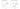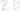## Multiclass Classification: One-vs-all Method

#### Multi-class classification

Now we will approach the classification of data when we have more than two categories. Instead of $y = \{0,1\}$ with two classes, we will expand our definition so that $y = \{0,1,...,k-1\}$ with $k$ classes.Examples of multi-class classification:

• Email tagging: Work, Friends, Family, Hobby
• Medical condition: Not ill, Cold, Flu
• Weather: Sunny, Cloudy, Rain, Snow

#### One-vs-all (One-vs-rest) methodWe divide our problem into $k$ binary classification problems. Then we train a Logistic regression classifier $h_\theta^{(i)}(x)$ for each class $i$ to predict the probability that $y=i$.

\begin{aligned}& y \in \lbrace0, 1, ..., k-1\rbrace \\& h_\theta^{(0)}(x) = P(y = 0 | x ; \theta) \\& h_\theta^{(1)}(x) = P(y = 1 | x ; \theta) \\& \cdots \\& h_\theta^{(k-1)}(x) = P(y = k-1 | x ; \theta) \end{aligned}

We are basically choosing one class and then lumping all the others into a single second class. We do this repeatedly, applying binary logistic regression to each case.

On a new input $x$, to make a prediction, pick the class $i$ that maximizes $h_\theta^{(i)}(x)$, i.e., use the hypothesis that returned the highest value as our prediction.

$\mathrm{prediction} = \max_i( h_\theta ^{(i)}(x) )$